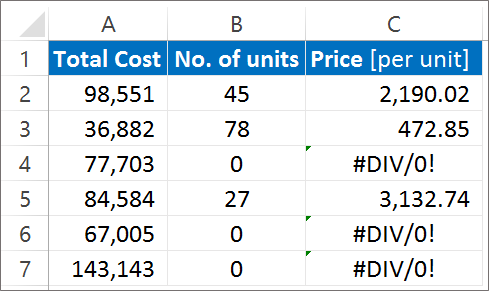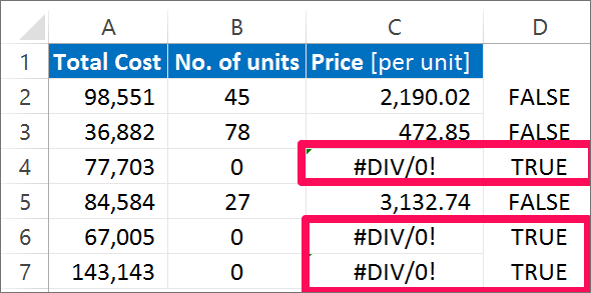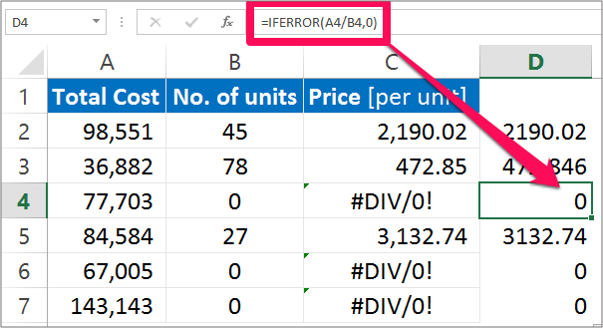Need Help? Chat with us

# ISERROR and IFERROR function in Excel

## ISERROR, IFERROR Function

Iserror, Iferror function in excel, We might experience some error while working in Excel. In this tutorial we will see how to use IFERROR, ISERROR Function in Excel in order to deal with the errors.

Let us take a look at these two function one after the other.

## Special Offer for First Time Users

If you are the first time user and want to learn Microsoft office skills, then you can avail this special offer discount on your email.### ISERROR Function

The Microsoft ISERROR FUNCTION is used to check for error values such as #N/A, #VALUE!, #REF!, #DIV/0!, #NUM!, #NAME? OR #NULL. This ISERROR is an in-built function in Excel categorized under Information Function. – iserror, iferror function

It is used as a worksheet function and also VBA function. As a worksheet function, ISERROR function is entered as a part of the formula. This function will return TRUE or FALSE. – iserror, iferror function

The ISERROR Function returns TRUE if there are any error value in the cell and FALSE if there are no error value in the cell. This function applies to all the version of Excel from 2000.

The Syntax used to calculate ISERROR function is:

ISERROR(value)

where,

Value: Here you enter the value that you want to test. If value is an Error value it will return TRUE otherwise it will return FALSE.

An Example below will help you to know about ISERROR function in Excel and how it works in Excel.

a. Below is the list of Material. We see error in the image below.b. Using the ISERROR formula to see if the cell contains Value error. The result will appear TRUE or FALSE.Here the cell D3 returns True Value as it the cell contains error. Also cell D6 and D7 return True value.

NOTE: There is difference between ISERR and ISERROR function in Excel. ISERR does not take #N/A error into consideration where ISERROR includes this error in the value.

ISERROR function can be utilized in many different ways. However it is most effective when combined and used with IF function. – iserror, iferror function

### IFERROR Function

IFERROR function is one of the Excels logical function. IF function consist of a logical test and it will return the value that we choose for it to return. The IFERROR Function provides us a method by which we can decide our further actions based on the either of the two outcomes. – iserror, iferror function

The main reason for using the IFERROR function in Excel is to trap error, handle those error and improve the experience of the users. The IFERROR function allows you to replace errors with another value or formula or expression whatever you specify. – iserror, iferror function

The syntax of IFERROR Function is:

IFERROR(value, value_if_error)

This function has two arguments

If the value argument is an error then return the value_if_error. IFERROR function is far more efficient that the ISERROR function.

Value_if_error is the value or formula or expression that you want IFERROR formula to return.

NOTE: Whatever value in enter in the value_if_arguments it will return that corresponding value.

This function applies to all the Excel version after 2007. It is a worksheet function and it returns some values as string or number etc. Based on our example Column C contains the formula to calculate the price per unit.

a. In our example, Cell C4, C6 and C7 contains errors.b. Column D in the spreadsheet will show you the result of IFERROR function. Enter the IFERROR formula as =IFERROR (A2/B2, O) and If the value argument contains numbers it will return number as the result. But, if the value contains error it will return 0 as the result.TIP: If you put (“”) in place of zero in the formula, the result will be a blank cell. It is though equivalent to zero.

Hope you like our tutorial on iserror, iferror function for more cool and amazing trick of excel Like our Excel Superstar Facebook Page and subscribe to our Excel Superstar YouTube channel. Excel Superstar is the leading online training company, which provides Online Excel Course in Hindi. Connect with us and become an Excel Superstar.